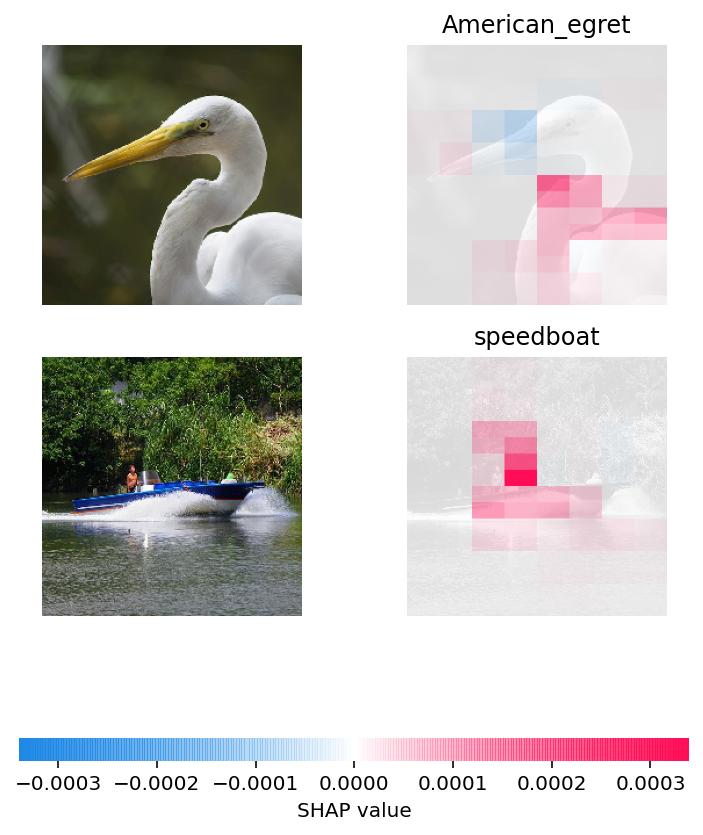# image plot

This notebook is designed to demonstrate (and so document) how to use the shap.plots.image function.

:

import json

from tensorflow.keras.applications.resnet50 import ResNet50, preprocess_input

import shap

# load pre-trained model and choose two images to explain
model = ResNet50(weights="imagenet")

def f(X):
tmp = X.copy()
preprocess_input(tmp)
return model(tmp)

X, y = shap.datasets.imagenet50()

# load the ImageNet class names as a vectorized mapping function from ids to names
url = "https://s3.amazonaws.com/deep-learning-models/image-models/imagenet_class_index.json"
with open(shap.datasets.cache(url)) as file:
class_names = [v for v in json.load(file).values()]

# define a masker that is used to mask out partitions of the input image, this one uses a blurred background

# By default the Partition explainer is used for all  partition explainer

# here we use 500 evaluations of the underlying model to estimate the SHAP values
shap_values = explainer(
X[1:3], max_evals=500, batch_size=50, outputs=shap.Explanation.argsort.flip[:1]
)
shap.image_plot(shap_values)

explainers.Partition is still in an alpha state, so use with caution...

Partition explainer: 3it [00:18,  6.10s/it]Have an idea for more helpful examples? Pull requests that add to this documentation notebook are encouraged!